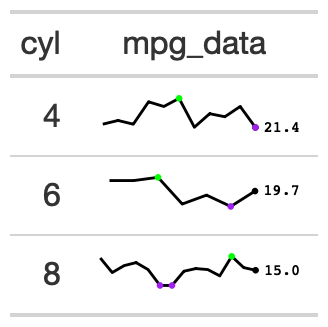The gt_plt_sparkline function takes an existing gt_tbl object and adds sparklines via the ggplot2. Note that if you'd rather plot summary distributions (ie density/histograms) you can instead use: gtExtras::gt_plt_dist()

## Usage

gt_plt_sparkline(
gt_object,
column,
type = "default",
fig_dim = c(5, 30),
palette = c("black", "black", "purple", "green", "lightgrey"),
same_limit = TRUE,
label = TRUE
)

## Arguments

gt_object

An existing gt table object of class gt_tbl

column

The column wherein the sparkline plot should replace existing data. Note that the data must be represented as a list of numeric values ahead of time.

type

A string indicating the type of plot to generate, accepts "default", "points", "shaded", "ref_median", 'ref_mean', "ref_iqr", "ref_last". "points" will add points to every observation instead of just the high/low and final. "shaded" will add shading below the sparkline. The "ref_" options add a thin reference line based off the summary statistic chosen

fig_dim

A vector of two numbers indicating the height/width of the plot in mm at a DPI of 25.4, defaults to c(5,30)

palette

A character string with 5 elements indicating the colors of various components. Order matters, and palette = sparkline color, final value color, range color low, range color high, and 'type' color (eg shading or reference lines). To show a plot with no points (only the line itself), use: palette = c("black", rep("transparent", 4)).

same_limit

A logical indicating that the plots will use the same axis range (TRUE) or have individual axis ranges (FALSE).

label

A logical indicating whether the sparkline will have a numeric label for the last value in the vector, placed at the end of the plot.

## Value

An object of class gt_tbl.

## Examples

 library(gt)
gt_sparkline_tab <- mtcars %>%
dplyr::group_by(cyl) %>%
# must end up with list of data for each row in the input dataframe
dplyr::summarize(mpg_data = list(mpg), .groups = "drop") %>%
gt() %>%
gt_plt_sparkline(mpg_data)

## Figures## Function ID

1-4

Other Plotting: gt_plt_bar_pct(), gt_plt_bar_stack(), gt_plt_bar(), gt_plt_dist(), gt_plt_percentile(), gt_plt_point(), gt_plt_winloss()